# Common fixed point theorem for a hybrid pair of mappings in Hausdorff fuzzy metric spaces

## Abstract

In this paper, we prove a coupled fixed point theorem for a multivalued fuzzy contraction mapping in complete Hausdorff fuzzy metric spaces. As an application of the first theorem, a coupled coincidence and coupled common fixed point theorem has been proved for a hybrid pair of multivalued and single-valued mappings. It is worth mentioning that to find coupled coincidence points, we do not employ the condition of continuity of any mapping involved therein. Also, coupled coincidence points are obtained without exploiting any type of commutativity condition. Our results extend, improve, and unify some well-known results in the literature.

MSC:47H10, 47H04, 47H07.

## 1 Introduction and preliminaries

Bhaskar and Lakshmikantham  introduced the concept of a coupled fixed point of a mapping F from $X×X$ to X and established some coupled fixed point theorems in partially ordered sets. Later on some authors gave improved and generalized results in this context. For details, we refer to [2, 3].

The concept of fuzzy sets was initiated by Zadeh  in 1965. Fuzzy metric spaces were introduced by Kramosil and Michalek . George and Veeramani [6, 7] modified the notion of fuzzy metric spaces by using continuous t-norm and generalized the concept of a probabilistic metric space to a fuzzy situation. Then a number of authors started the study of fixed point theory in fuzzy metric spaces; for a detailed survey, we refer to  and the references therein. Recently López and Romaguera  introduced a Hausdorff fuzzy metric on a set of nonempty compact subsets of a given fuzzy metric space. In 2011, Kiany et al.  proved fixed point and endpoint theorems for set-valued fuzzy contraction maps in fuzzy metric spaces.

Recently Abbas  introduced the concept of coupled fixed points of a mapping $F:X×X\to {2}^{X}$ (a collection of all nonempty subsets of X) and coupled coincidence points of a hybrid pair F and $g:X\to X$. The aim of this paper is to obtain a coupled fixed point theorem for F and a coupled coincidence and coupled common fixed point theorem for a hybrid pair $\left\{F,g\right\}$ which satisfies a contractive condition in complete Hausdorff fuzzy metric spaces. It is to be noted that to find coupled coincidence points, we do not employ the condition of commutativity and continuity of any mapping involved therein. Our results unify, extend, and generalize various known comparable results given in existing literature (see, for example,  and some references therein).

Definition 1 

A binary operation $\ast :{\left[0,1\right]}^{2}\to \left[0,1\right]$ is called a continuous t-norm if

1. (1)

is associative and commutative;

2. (2)

is continuous;

3. (3)

$a\ast 1=a$ for all $a\in \left[0,1\right]$;

4. (4)

$a\ast b\le c\ast d$ whenever $a\le c$ and $b\le d$.

Definition 2 

Let X be a nonempty set and be a continuous t-norm. If a mapping $M:{X}^{2}×\left[0,\mathrm{\infty }\right)\to \left[0,1\right]$ satisfies the following conditions:

(F1) $M\left(x,y,t\right)>0$;

(F2) $M\left(x,y,t\right)=1$ if and only if $x=y$;

(F3) $M\left(x,y,t\right)=M\left(y,x,t\right)$;

(F4) $M\left(x,y,t\right)\ast M\left(y,z,s\right)\le M\left(x,z,t+s\right)$;

(F5) $M\left(x,y,t\right):\left(0,\mathrm{\infty }\right)\to \left[0,1\right]$ is continuous;

for each $x,y,z\in X$ and $s,t>0$, then 3-tuple $\left(X,M,\ast \right)$ is called a fuzzy metric space.

Example 3 

Let $\left(X,d\right)$ be a metric space. Define $a\ast b=min\left\{a,b\right\}$ and

$M\left(x,y,t\right)=\frac{t}{t+d\left(x,y\right)}$

for all $x,y\in X$ and $t>0$. Then $\left(X,M,\ast \right)$ is a fuzzy metric space. We call this a fuzzy metric M, the standard fuzzy metric induced by d.

Definition 4 

Let $\left(X,M,\ast \right)$ be a fuzzy metric space.

1. (i)

A sequence $\left\{{x}_{n}\right\}$ is said to be convergent to a point $x\in X$ if ${lim}_{n\to \mathrm{\infty }}M\left({x}_{n},x,t\right)=1$ for all $t>0$.

2. (ii)

A sequence $\left\{{x}_{n}\right\}$ is said to be a Cauchy sequence if ${lim}_{n\to \mathrm{\infty }}M\left({x}_{m},{x}_{n},t\right)=1$ for all $t>0$.

3. (iii)

A fuzzy metric space in which every Cauchy sequence is convergent is said to be complete.

4. (iv)

A subset $A\subseteq X$ is said to be closed if for each convergent sequence $\left\{{x}_{n}\right\}$ with ${x}_{n}\in A$ and ${x}_{n}\to x$, we have $x\in A$.

5. (v)

A subset $A\subseteq X$ is said to be compact if each sequence in A has a convergent subsequence. The set of all compact subsets of X will be denoted by $K\left(X\right)$.

Lemma 5 

For all $x,y\in X$, $M\left(x,y,\cdot \right)$ is nondecreasing.

Definition 6 Let $\left(M,X,\ast \right)$ be a fuzzy metric space, M is said to be continuous on ${X}^{2}×\left(0,\mathrm{\infty }\right)$ if

$\underset{n\to \mathrm{\infty }}{lim}M\left({x}_{n},{y}_{n},{t}_{n}\right)=M\left(x,y,t\right),$

whenever $\left\{\left({x}_{n},{y}_{n},{t}_{n}\right)\right\}$ is a sequence in ${X}^{2}×\left(0,\mathrm{\infty }\right)$ which converges to a point $\left(x,y,t\right)\in {X}^{2}×\left(0,\mathrm{\infty }\right)$; that is,

$\underset{n\to \mathrm{\infty }}{lim}M\left({x}_{n},x,t\right)=\underset{n\to \mathrm{\infty }}{lim}M\left({y}_{n},y,t\right)=1\phantom{\rule{1em}{0ex}}\text{and}\phantom{\rule{1em}{0ex}}\underset{n\to \mathrm{\infty }}{lim}M\left(x,y,{t}_{n}\right)=M\left(x,y,t\right).$

Lemma 7 

M is a continuous function on ${X}^{2}×\left(0,\mathrm{\infty }\right)$.

Kiany et al.  introduced the following lemma in fuzzy metric spaces.

Lemma 8 

Let $\left(X,M,\ast \right)$ be a fuzzy metric space satisfying

$\underset{n\to \mathrm{\infty }}{lim}{\ast }_{i=n}^{\mathrm{\infty }}M\left(x,y,t{h}^{i}\right)=1$
(1)

for every $x,y\in X$, $t>0$, and $h>1$. Suppose $\left\{{x}_{n}\right\}$ is a sequence in X satisfying

$M\left({x}_{n},{x}_{n+1},\alpha t\right)\ge M\left({x}_{n-1},{x}_{n},t\right)$

for all $n\in \mathbb{N}$ and $0<\alpha <1$. Then $\left\{{x}_{n}\right\}$ is a Cauchy sequence.

Lemma 9 

Let $\left(X,M,\ast \right)$ be a fuzzy metric space. Then, for each $a\in X$, $B\in K\left(X\right)$, and $t>0$, there is a ${b}_{0}\in B$ such that $M\left(a,B,t\right)=M\left(a,{b}_{0},t\right)$, where

$M\left(a,B,t\right)=\underset{b\in B}{sup}M\left(a,b,t\right).$

Definition 10 

Let $\left(X,M,\ast \right)$ be a fuzzy metric space. For each $A,B\in K\left(X\right)$ and $t>0$, set

${H}_{M}\left(A,B,t\right)=min\left\{\underset{x\in A}{inf}M\left(x,B,t\right),\underset{y\in B}{inf}M\left(A,y,t\right)\right\}.$

The 3-tuple $\left(K\left(X\right),{H}_{M},\ast \right)$ is called a Hausdorff fuzzy metric space.

Lemma 11 

Let X be a nonempty set and $g:X\to X$ be a mapping. Then there exists a subset $E\subseteq X$ such that $g\left(E\right)=g\left(X\right)$ and $g:E\to X$ is one-to-one.

Theorem 12 

Let $\left(X,M,\ast \right)$ be a complete fuzzy metric. Suppose $F:X×X\to K\left(X\right)$ is a multivalued mapping such that

${H}_{M}\left(Fx,Fy,\varphi \left(d\left(x,y,t\right)\right)t\right)\ge M\left(x,y,t\right),$

for each $x,y\in X$ and $t>0$, where $\varphi :\left[0,\mathrm{\infty }\right)\to \left[0,1\right]$ satisfying ${lim sup}_{r\to {t}^{+}}\varphi \left(r\right)<1$, for all $t\in \left[0,\mathrm{\infty }\right)$, and $d\left(x,y,t\right)=\frac{t}{M\left(x,y,t\right)}-t$. Furthermore, assume that $\left(X,M,\ast \right)$ satisfies (1) for some ${x}_{0}$ and ${x}_{1}\in F\left({x}_{0}\right)$. Then F has a fixed point.

We also need the following definitions given in .

Definition 13 

Let X be a nonempty set, $F:X×X\to {2}^{X}$ (a collection of all nonempty subsets of X) and $g:X\to X$. An element $\left(x,y\right)\in X×X$ is called

(C1) a coupled fixed point of F if $x\in F\left(x,y\right)$ and $y\in F\left(y,x\right)$;

(C2) a coupled coincidence point of a hybrid pair $\left\{F,g\right\}$ if $g\left(x\right)\in F\left(x,y\right)$ and $g\left(y\right)\in F\left(y,x\right)$;

(C3) a coupled common fixed point of a hybrid pair $\left\{F,g\right\}$ if $x=g\left(x\right)\in F\left(x,y\right)$ and $y=g\left(y\right)\in F\left(y,x\right)$.

We denote the set of coupled coincidence points of mappings F and g by $C\left(F,g\right)$. Note that if $\left(x,y\right)\in C\left(F,g\right)$, then $\left(y,x\right)$ is also in $C\left(F,g\right)$.

Definition 14 

Let $F:X×X\to {2}^{X}$ be a multivalued mapping and g be a self-map on X. The hybrid pair $\left\{F,g\right\}$ is called w-compatible if $g\left(F\left(x,y\right)\right)\subseteq F\left(gx,gy\right)$ whenever $\left(x,y\right)\in C\left(F,g\right)$.

Definition 15 

Let $F:X×X\to {2}^{X}$ be a multivalued mapping and g be a self-mapping on X. The mapping g is called F-weakly commuting at some point $\left(x,y\right)\in X×X$ if ${g}^{2}\left(x\right)\in F\left(gx,gy\right)$ and ${g}^{2}\left(y\right)\in F\left(gy,gx\right)$.

## 2 Coupled fixed and coincidence point theorems

In the following theorem, we obtain a coupled fixed point for a multivalued mapping satisfying a contractive condition.

Theorem 16 Let $\left(X,M,\ast \right)$ be a complete fuzzy metric space and let $F:X×X\to K\left(X\right)$ be a set-valued mapping satisfying

${H}_{M}\left(F\left(x,y\right),F\left(u,v\right),\varphi \left(d\left(x,u,t\right)\right)t\right)\ge min\left\{M\left(x,u,t\right),M\left(x,F\left(x,y\right),t\right)\right\}$
(2)

for each $x,y,u,v\in X$, $t>0$. Suppose that $d\left(x,u,t\right)=\frac{t}{M\left(x,u,t\right)}-t$ and $\varphi :\left[0,\mathrm{\infty }\right)\to \left[0,1\right)$ is a mapping satisfying

$\underset{r\to {t}^{+}}{lim sup}\varphi \left(r\right)<1$

for all $t\in \left[0,\mathrm{\infty }\right)$. Furthermore, assume that $\left(X,M,\ast \right)$ satisfies (1) for some ${x}_{0},{x}_{1}\in F\left({x}_{0},{y}_{0}\right)$ and ${y}_{0},{y}_{1}\in F\left({y}_{0},{x}_{0}\right)$. Then F has a coupled fixed point.

Proof Let ${x}_{0},{y}_{0}\in X$ be arbitrary. Choose ${x}_{1}\in F\left({x}_{0},{y}_{0}\right)$ and ${y}_{1}\in F\left({y}_{0},{x}_{0}\right)$. Since F is compact valued, then by Lemma 9 there exists ${x}_{2}\in F\left({x}_{1},{y}_{1}\right)$ such that

$\begin{array}{rcl}M\left({x}_{1},{x}_{2},t\right)& \ge & M\left({x}_{1},{x}_{2},\varphi \left(d\left({x}_{0},{x}_{1},t\right)\right)t\right)\\ =& \underset{y\in F\left({x}_{1},{y}_{1}\right)}{sup}M\left({x}_{1},y,\varphi \left(d\left({x}_{0},{x}_{1},t\right)\right)t\right)\\ \ge & {H}_{M}\left(F\left({x}_{0},{y}_{0}\right),F\left({x}_{1},{y}_{1}\right),\varphi \left(d\left({x}_{0},{x}_{1},t\right)\right)t\right)\\ \ge & min\left\{M\left({x}_{0},{x}_{1},t\right),M\left({x}_{0},F\left({x}_{0},{y}_{0}\right),t\right)\right\}\ge M\left({x}_{0},{x}_{1},t\right).\end{array}$

Since F is compact valued, there exists ${y}_{2}\in F\left({y}_{1},{x}_{1}\right)$ such that

$\begin{array}{rcl}M\left({y}_{1},{y}_{2},t\right)& \ge & M\left({y}_{1},{y}_{2},\varphi \left(d\left({y}_{0},{y}_{1},t\right)\right)t\right)\\ =& \underset{y\in F\left({y}_{1},{x}_{1}\right)}{sup}M\left({y}_{1},y,\varphi \left(d\left({y}_{0},{y}_{1},t\right)\right)t\right)\\ \ge & {H}_{M}\left(F\left({y}_{0},{x}_{0}\right),F\left({y}_{1},{x}_{1}\right),\varphi \left(d\left({y}_{0},{y}_{1},t\right)\right)t\right)\\ \ge & min\left\{M\left({y}_{0},{y}_{1},t\right),M\left({y}_{0},F\left({y}_{0},{x}_{0}\right),t\right)\right\}\ge M\left({y}_{0},{y}_{1},t\right).\end{array}$

Continuing this process, we obtain a sequence ${\left\{{x}_{n}\right\}}_{n\ge 0}$ and ${\left\{{y}_{n}\right\}}_{n\ge 0}$ in X such that ${x}_{n+1}\in F\left({x}_{n},{y}_{n}\right)$ and ${y}_{n+1}\in F\left({y}_{n},{x}_{n}\right)$ satisfying

$\begin{array}{rcl}M\left({x}_{n},{x}_{n+1},t\right)& \ge & M\left({x}_{n},{x}_{n+1},\varphi \left(d\left({x}_{n-1},{x}_{n},t\right)\right)t\right)\\ =& \underset{y\in F\left({x}_{n},{y}_{n}\right)}{sup}M\left({x}_{n},y,\varphi \left(d\left({x}_{n-1},{x}_{n},t\right)\right)t\right)\\ \ge & {H}_{M}\left(F\left({x}_{n-1},{y}_{n-1}\right),F\left({x}_{n},{y}_{n}\right),\varphi \left(d\left({x}_{n-1},{x}_{n},t\right)\right)t\right)\\ \ge & min\left\{M\left({x}_{n-1},{x}_{n},t\right),M\left({x}_{n-1},F\left({x}_{n-1},{y}_{n-1}\right),t\right)\right\}\ge M\left({x}_{n-1},{x}_{n},t\right).\end{array}$

That is, we obtain

$M\left({x}_{n},{x}_{n+1},t\right)\ge M\left({x}_{n-1},{x}_{n},t\right).$
(3)

Similarly,

$M\left({y}_{n},{y}_{n+1},t\right)\ge M\left({y}_{n-1},{y}_{n},t\right).$
(4)

Inequalities (3) and (4) show that the sequences ${\left\{M\left({x}_{n},{x}_{n+1},t\right)\right\}}_{n}$ and ${\left\{M\left({y}_{n},{y}_{n+1},t\right)\right\}}_{n}$ are nondecreasing. Thus, $d\left({x}_{n},{x}_{n+1},t\right)$ and $d\left({y}_{n},{y}_{n+1},t\right)$ are nonnegative nonincreasing and so they are convergent, say, to ${l}_{1}\ge 0$ and ${l}_{2}\ge 0$. Since, by the given assumption,

$\begin{array}{r}\underset{n\to \mathrm{\infty }}{lim sup}\varphi \left(d\left({x}_{n},{x}_{n+1},t\right)\right)\le \underset{r\to {l}_{1}^{+}}{lim sup}\varphi \left(r\right)<1\phantom{\rule{1em}{0ex}}\text{and}\\ \underset{n\to \mathrm{\infty }}{lim sup}\varphi \left(d\left({y}_{n},{y}_{n+1},t\right)\right)\le \underset{r\to {l}_{2}^{+}}{lim sup}\varphi \left(r\right)<1,\end{array}$
(5)

then there exist ${k}_{1}<1$, ${k}_{2}<1$, and ${N}_{1},{N}_{2}\in \mathbb{N}$ such that

(6)

Since $M\left(x,y,\cdot \right)$ is nondecreasing, then (3), (4), and (6) yield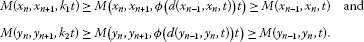Then we obtain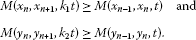Hence, by Lemma 8, $\left\{{x}_{n}\right\}$ and $\left\{{y}_{n}\right\}$ are Cauchy sequences. Since $\left(X,M,\ast \right)$ is a complete fuzzy metric space, then there exist x and y in X such that ${lim}_{n\to \mathrm{\infty }}{x}_{n}=x$ and ${lim}_{n\to \mathrm{\infty }}{y}_{n}=y$, we have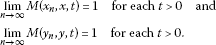Thus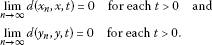Since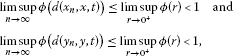then there exist ${\lambda }_{1}$, ${\lambda }_{2}$ such that ${\lambda }_{1}<1$, ${\lambda }_{2}<1$, and ${n}_{1},{n}_{2}\in \mathbb{N}$ such that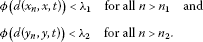Now, we show that $x\in F\left(x,y\right)$ and $y\in F\left(y,x\right)$. Consider

$\begin{array}{rcl}{H}_{M}\left(F\left({x}_{n},{y}_{n}\right),F\left(x,y\right),{\lambda }_{1}t\right)& \ge & {H}_{M}\left(F\left({x}_{n},{y}_{n}\right),F\left(x,y\right),\varphi \left(d\left({x}_{n},x,t\right)\right)t\right)\\ \ge & min\left\{M\left({x}_{n},x,t\right),M\left({x}_{n},F\left({x}_{n},{y}_{n}\right),t\right)\right\}\\ \ge & min\left\{M\left({x}_{n},x,t\right),M\left({x}_{n},{x}_{n+1},t\right)\right\}\\ \ge & min\left\{M\left({x}_{n},x,t\right),M\left({x}_{n},x,\frac{t}{2}\right)\ast M\left(x,{x}_{n+1},\frac{t}{2}\right)\right\}.\end{array}$

On taking limit as $n\to \mathrm{\infty }$, we obtain

$\underset{n\to \mathrm{\infty }}{lim}{H}_{M}\left(F\left({x}_{n},{y}_{n}\right),F\left(x,y\right),{\lambda }_{1}t\right)=1.$

So that

$\underset{n\to \mathrm{\infty }}{lim}{H}_{M}\left(F\left({x}_{n},{y}_{n}\right),F\left(x,y\right),t\right)=1.$
(7)

Similarly, we can obtain

$\underset{n\to \mathrm{\infty }}{lim}{H}_{M}\left(F\left({y}_{n},{x}_{n}\right),F\left(y,x\right),t\right)=1.$
(8)

Since ${x}_{n+1}\in F\left({x}_{n},{y}_{n}\right)$ and ${y}_{n+1}\in F\left({y}_{n},{x}_{n}\right)$, from (7) and (8) we obtain

$\underset{n\to \mathrm{\infty }}{lim}\underset{a\in F\left(x,y\right)}{sup}M\left({x}_{n+1},a,t\right)=1\phantom{\rule{1em}{0ex}}\text{and}\phantom{\rule{1em}{0ex}}\underset{n\to \mathrm{\infty }}{lim}\underset{b\in F\left(y,x\right)}{sup}M\left({y}_{n+1},b,t\right)=1.$

There exist sequences ${w}_{n}\in F\left(x,y\right)$ and ${z}_{n}\in F\left(y,x\right)$ such that

$\underset{n\to \mathrm{\infty }}{lim}M\left({x}_{n+1},{w}_{n+1},t\right)=1\phantom{\rule{1em}{0ex}}\text{and}\phantom{\rule{1em}{0ex}}\underset{n\to \mathrm{\infty }}{lim}M\left({y}_{n+1},{z}_{n+1},t\right)=1$

for each $t>0$. Now, for each $n\in \mathbb{N}$, we have

$M\left({w}_{n+1},x,t\right)\ge M\left({w}_{n+1},{x}_{n+1},\frac{t}{2}\right)\ast M\left({x}_{n+1},x,\frac{t}{2}\right).$

On taking limit as $n\to \mathrm{\infty }$, we get

$\underset{n\to \mathrm{\infty }}{lim}M\left({w}_{n+1},x,t\right)=1.$
(9)

Similarly, for each $n\in \mathbb{N}$, we have

$M\left({z}_{n+1},y,t\right)\ge M\left({z}_{n+1},{y}_{n+1},\frac{t}{2}\right)\ast M\left({y}_{n+1},y,\frac{t}{2}\right).$

On taking limit as $n\to \mathrm{\infty }$, we get

$\underset{n\to \mathrm{\infty }}{lim}M\left({z}_{n+1},y,t\right)=1.$
(10)
1. (9)

and (10) imply

$\underset{n\to \mathrm{\infty }}{lim}{w}_{n}=x\phantom{\rule{1em}{0ex}}\text{and}\phantom{\rule{1em}{0ex}}\underset{n\to \mathrm{\infty }}{lim}{z}_{n}=y.$

Since $F\left(x,y\right)$ and $F\left(y,x\right)$ are compact, we get $x\in F\left(x,y\right)$ and $y\in F\left(y,x\right)$. □

Corollary 17 Let $\left(X,M,\ast \right)$ be a complete fuzzy metric space and let $F:X×X\to K\left(X\right)$ be a mapping satisfying

${H}_{M}\left(F\left(x,y\right),F\left(u,v\right),kt\right)\ge min\left\{M\left(x,u,t\right),M\left(x,F\left(x,y\right),t\right)\right\}$
(11)

for each $x,y,u,v\in X$, $t>0$, and $0. Suppose that $\left(X,M,\ast \right)$ satisfies (1) for some ${x}_{0},{x}_{1}\in F\left({x}_{0},{y}_{0}\right)$ and ${y}_{0},{y}_{1}\in F\left({y}_{0},{x}_{0}\right)$. Then F has a coupled fixed point.

Corollary 18 Let $\left(X,M,\ast \right)$ be a complete fuzzy metric space and let $F:X×X\to K\left(X\right)$ be a set-valued mapping satisfying

${H}_{M}\left(F\left(x,y\right),F\left(u,v\right),\varphi \left(d\left(x,u,t\right)\right)t\right)\ge M\left(x,u,t\right)$

for each $x,y,u,v\in X$, $t>0$. Suppose that $d\left(x,u,t\right)=\frac{t}{M\left(x,u,t\right)}-t$ and $\varphi :\left[0,\mathrm{\infty }\right)\to \left[0,1\right)$ is a mapping satisfying

$\underset{r\to {t}^{+}}{lim sup}\varphi \left(r\right)<1$

for all $t\in \left[0,\mathrm{\infty }\right)$. Furthermore, assume that $\left(X,M,\ast \right)$ satisfies (1) for some ${x}_{0},{x}_{1}\in F\left({x}_{0},{y}_{0}\right)$ and ${y}_{0},{y}_{1}\in F\left({y}_{0},{x}_{0}\right)$. Then F has a coupled fixed point.

Corollary 19 Let $\left(X,M,\ast \right)$ be a complete fuzzy metric space and let $F:X×X\to K\left(X\right)$ be a mapping satisfying

${H}_{M}\left(F\left(x,y\right),F\left(u,v\right),kt\right)\ge M\left(x,u,t\right)$

for each $x,y,u,v\in X$, $t>0$, and $0. Suppose that $\left(X,M,\ast \right)$ satisfies (1) for some ${x}_{0},{x}_{1}\in F\left({x}_{0},{y}_{0}\right)$ and ${y}_{0},{y}_{1}\in F\left({y}_{0},{x}_{0}\right)$. Then F has a coupled fixed point.

Example 20 Let $X=\left\{0,1,2\right\}$ and $d:X×X\to R$ be defined as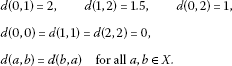Hence, $\left(X,d\right)$ is a metric space. Consider

$M\left(x,y,t\right)=\frac{t}{t+d\left(x,y\right)}$

for $t>0$ and $\left(X,M,\ast \right)$ satisfies (1). Define $F:X×X\to CB\left(X\right)$ as follows:

For $x\in \left\{0,2\right\}$ and $u\in \left\{0,2\right\}$ and for $x=1$ and $u=1$, we have

${H}_{M}\left(F\left(x,y\right),F\left(u,v\right),kt\right)=1$

for any $k\in \left(0,1\right)$. Hence, (11) is satisfied. For the rest of cases,

${H}_{M}\left(F\left(x,y\right),F\left(u,v\right),kt\right)=\frac{kt}{kt+1}$

and

$min\left\{M\left(x,u,t\right),M\left(x,F\left(x,y\right),t\right)\right\}\in \left\{0,\frac{t}{t+2},\frac{t}{t+1.5}\right\}.$

Hence, for all $x,y,u,v\in X$, $t>0$, and $k=\frac{2}{3}$, (11) holds. All the conditions of Corollary 17 and Theorem 16 with $\varphi \left(t\right)=k$ are satisfied. Moreover, $\left(0,0\right)$ and $\left(2,2\right)$ are coupled fixed points of F.

Example 21 Let $X=\left[0,1\right]$ be endowed with the usual metric $d\left(x,y\right)=|x-y|$ and $F\left(x,y\right)=\left[ln\left(1+x\right),1\right]$ for each $x,y\in X$. Let $M\left(x,y,t\right)=\frac{t}{t+d\left(x,y\right)}$ and let $\varphi \left(t\right)=\frac{ln\left(1+t\right)}{t}$ for each $t>0$. Then we have

$\begin{array}{rcl}{H}_{M}\left(F\left(x,y\right),F\left(u,v\right),\varphi \left(t\right)t\right)& =& {H}_{M}\left(F\left(x,y\right),F\left(u,v\right),t\right)\\ \ge & \frac{t}{t+|ln\left(1+u\right)-ln\left(1+x\right)|}\\ \ge & \frac{t}{t+|u-x|}\\ =& M\left(x,u,t\right)\end{array}$

for each $x,y\in X$ and $t>0$. Then by Corollary 18, F has a coupled fixed point ($\left(0,0\right)$ is a coupled fixed point of F).

Example 22 Let $X=R$ be endowed with the usual metric $d\left(x,y\right)=|x-y|$ and let $f\left(x,y\right)=\frac{1}{2}x$ for each $x,y\in X$. Let $M\left(x,y,t\right)=\frac{t}{t+d\left(x,y\right)}$ and let $\varphi \left(t\right)=\frac{1}{2}$ for each $t>0$. Then we have

$\begin{array}{rcl}{H}_{M}\left(f\left(x,y\right),f\left(u,v\right),\frac{1}{2}t\right)& =& M\left(f\left(x,y\right),f\left(u,v\right),\frac{1}{2}t\right)\\ =& M\left(\frac{1}{2}x,\frac{1}{2}u,\frac{1}{2}t\right)=M\left(x,u,t\right)\end{array}$

for each $x,y\in X$ and $t>0$. Then by Corollary 19, f has a coupled fixed point ($\left(0,0\right)$ is a coupled fixed point of f).

Now, as an application of the above theorem, we obtain a coupled coincidence and common fixed point theorem for a hybrid pair of multivalued and single-valued mappings.

Theorem 23 Let $\left(X,M,\ast \right)$ be a complete fuzzy metric space and let $F:X×X\to K\left(X\right)$ and $g:X\to X$ be mappings satisfying

${H}_{M}\left(F\left(x,y\right),F\left(u,v\right),\varphi \left(d\left(x,u,t\right)\right)t\right)\ge min\left\{M\left(gx,gu,t\right),M\left(gx,F\left(gx,gy\right),t\right)\right\}$
(12)

for each $x,y,u,v\in X$, $t>0$. Suppose that $d\left(x,u,t\right)=\frac{t}{M\left(x,u,t\right)}-t$ and $\varphi :\left[0,\mathrm{\infty }\right)\to \left[0,1\right)$ is a mapping satisfying

$\underset{r\to {t}^{+}}{lim sup}\varphi \left(r\right)<1$

for all $t\in \left[0,\mathrm{\infty }\right)$. Furthermore, assume that $F\left(X×X\right)\subseteq g\left(X\right)$ and $\left(X,M,\ast \right)$ satisfies (1) for some $g{x}_{0},g{x}_{1}\in F\left({x}_{0},{y}_{0}\right)$ and $g{y}_{0},g{y}_{1}\in F\left({y}_{0},{x}_{0}\right)$. Then F and g have a coupled coincidence point. Moreover, F and g have a coupled common fixed point if one of the following conditions holds:

1. (a)

F and g are w-compatible, ${lim}_{n\to \mathrm{\infty }}{g}^{n}x=u$ and ${lim}_{n\to \mathrm{\infty }}{g}^{n}y=v$ for some $\left(x,y\right)\in C\left(F,g\right)$, $u,v\in X$, and g is continuous at u and v.

2. (b)

g is F-weakly commuting for some $\left(x,y\right)\in C\left(g,F\right)$, and gx and gy are fixed points of g, that is, ${g}^{2}x=gx$ and ${g}^{2}y=gy$.

3. (c)

g is continuous at x, y for some $\left(x,y\right)\in C\left(g,F\right)$ and for some $u,v\in X$, ${lim}_{n\to \mathrm{\infty }}{g}^{n}u=x$ and ${lim}_{n\to \mathrm{\infty }}{g}^{n}v=y$.

Proof By Lemma 11 there exists $E\subseteq X$ such that $g:E\to X$ is one-to-one and $g\left(E\right)=g\left(X\right)$. Now, define a mapping $\mathcal{A}:g\left(E\right)×g\left(E\right)\to K\left(X\right)$ by

(13)

and since g is one-one on E, so $\mathcal{A}$ is well defined. Further,

$\begin{array}{rcl}{H}_{M}\left(\mathcal{A}\left(gx,gy\right),\mathcal{A}\left(gu,gv\right),\varphi \left(d\left(x,u,t\right)\right)t\right)& =& {H}_{M}\left(F\left(x,y\right),F\left(u,v\right),\varphi \left(d\left(x,u,t\right)\right)t\right)\\ \ge & min\left\{M\left(gx,gu,t\right),M\left(gx,F\left(gx,gy\right),t\right)\right\}.\end{array}$
(14)

Hence, $\mathcal{A}$ satisfies (2) and all the conditions of Theorem 16. By using Theorem 16 with a mapping $\mathcal{A}$, it follows that $\mathcal{A}$ has a coupled fixed point $\left(u,v\right)\in g\left(E\right)×g\left(E\right)$. Finally, it is left to prove that F and g have a coupled coincidence point. Since $\mathcal{A}$ has a coupled fixed point $\left(u,v\right)\in g\left(E\right)×g\left(E\right)$, we get

$u\in \mathcal{A}\left(u,v\right),\phantom{\rule{1em}{0ex}}\text{and}\phantom{\rule{1em}{0ex}}v\in \mathcal{A}\left(v,u\right).$
(15)

Since $F\left(X×X\right)\subseteq g\left(X\right)$, so there exist ${u}_{1},{v}_{1}\in X×X$ such that $g{u}_{1}=u$ and $g{v}_{1}=v$. Thus, it follows from (15),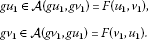This implies that $\left({u}_{1},{v}_{1}\right)\in$ $X×X$ is a coupled coincidence point of F and g. Hence, $C\left(F,g\right)$ is nonempty. Suppose now that (a) holds. Then, for some $\left(x,y\right)\in C\left(F,g\right)$,

$\underset{n\to \mathrm{\infty }}{lim}{g}^{n}x=u\phantom{\rule{1em}{0ex}}\text{and}\phantom{\rule{1em}{0ex}}\underset{n\to \mathrm{\infty }}{lim}{g}^{n}y=v,$

where $u,v\in X$. Since g is continuous at u and v, we have that u and v are fixed points of g. As F and g are w-compatible, so

That is, for all $n\ge 1$,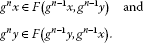Using (12), we obtain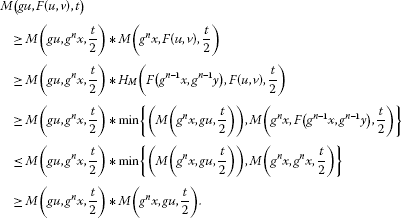On taking limit as $n\to \mathrm{\infty }$, we get

$\begin{array}{rcl}M\left(gu,F\left(u,v\right),t\right)& \ge & M\left(u,u,\frac{t}{2}\right)\ast M\left(u,u,\frac{t}{2}\right)\\ \ge & 1\ast 1=1.\end{array}$

This implies $gu\in F\left(u,v\right)$. Similarly, $gv\in F\left(v,u\right)$. Consequently, $u=gu\in F\left(u,v\right)$ and $v=gv\in F\left(v,u\right)$. Hence, $\left(u,v\right)$ is a coupled common fixed point of F and g. Suppose now that (b) holds. If for some $\left(x,y\right)\in C\left(F,g\right)$, g is F-commuting and ${g}^{2}x=gx$ and ${g}^{2}y=gy$, then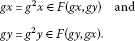Hence, $\left(gx,gy\right)$ is a coupled common fixed point of F and g. Suppose now that (c) holds and assume that for some $\left(x,y\right)\in C\left(g,F\right)$ and for some $u,v\in X$, ${lim}_{n\to \mathrm{\infty }}{g}^{n}u=x$ and ${lim}_{n\to \mathrm{\infty }}{g}^{n}v=y$. By the continuity of g at x and y, we get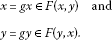Hence, $\left(x,y\right)$ is a coupled common fixed point of F and g. □

## References

1. Bhashkar TG, Lakshmikantham V: Fixed point theorems in partially ordered metric spaces and applications. Nonlinear Anal. TMA 2006, 65: 1379–1393. 10.1016/j.na.2005.10.017

2. Lakshmikantham V, Ćirić L: Coupled fixed point theorems for nonlinear contractions in partially ordered metric space. Nonlinear Anal. TMA 2009, 70: 4341–4349. 10.1016/j.na.2008.09.020

3. Sabetghadam F, Masiha HP, Sanatpour AH: Some coupled fixed point theorems in cone metric space. Fixed Point Theory Appl. 2009., 2009: Article ID 125426

4. Zadeh LA: Fuzzy sets. Inf. Control 1965, 8: 103–112.

5. Kramosil I, Michalek J: Fuzzy metric and statistical metric spaces. Kybernetika 1975, 11: 326–334.

6. George A, Veeramani P: On some results in fuzzy metric spaces. Fuzzy Sets Syst. 1994, 64: 395–399. 10.1016/0165-0114(94)90162-7

7. George A, Veeramani P: On some results of analysis for fuzzy metric spaces. Fuzzy Sets Syst. 1997, 90: 365–368. 10.1016/S0165-0114(96)00207-2

8. Ray AD, Saha PK: Fixed point theorems on generalized fuzzy metric spaces. Hacet. J. Math. Stat. 2010, 39: 1–9.

9. Fang JX: On fixed point theorems in fuzzy metric spaces. Fuzzy Sets Syst. 1992, 46: 107–113. 10.1016/0165-0114(92)90271-5

10. Grabiec M: Fixed points in fuzzy metric spaces. Fuzzy Sets Syst. 1983, 27: 385–389.

11. Gregori V, Sapena A: On fixed-point theorems in fuzzy metric spaces. Fuzzy Sets Syst. 2002, 125: 245–252. 10.1016/S0165-0114(00)00088-9

12. Liu Y, Li Z: Coincidence point theorems in probabilistic and fuzzy metric spaces. Fuzzy Sets Syst. 2007, 158: 58–70. 10.1016/j.fss.2006.07.010

13. Miheţ D: On the existence and the uniqueness of fixed points of Sehgal contractions. Fuzzy Sets Syst. 2005, 156: 135–141. 10.1016/j.fss.2005.05.024

14. Miheţ D: On fuzzy contractive mappings in fuzzy metric spaces. Fuzzy Sets Syst. 2007, 158: 915–921. 10.1016/j.fss.2006.11.012

15. Razani A: A contraction theorem in fuzzy metric space. Fixed Point Theory Appl. 2005, 3: 257–265.

16. Sedghi S, Altun I, Shobe N: Coupled fixed point theorems for contractions in fuzzy metric spaces. Nonlinear Anal. 2010, 72: 1298–1304. 10.1016/j.na.2009.08.018

17. Som T, Mukherjee RN: Some fixed point theorems for fuzzy mappings. Fuzzy Sets Syst. 1989, 33: 213–219. 10.1016/0165-0114(89)90242-X

18. Žikić T: On fixed point theorems of Gregori and Sapena. Fuzzy Sets Syst. 2004, 144: 421–429. 10.1016/S0165-0114(03)00179-9

19. Rodríguez-López J, Romaguera S: The Hausdorff fuzzy metric on compact sets. Fuzzy Sets Syst. 2004, 147: 273–283. 10.1016/j.fss.2003.09.007

20. Kiany F, Amini-Harandi A: Fixed point and endpoint theorems for set-valued fuzzy contraction maps in fuzzy metric spaces. Fixed Point Theory Appl. 2011., 2011: Article ID 94. doi:10.1186/1687–1812–2011–94

21. Abbas M, Ćirić L, Damjanović B, Khan MA: Coupled coincidence and common fixed point theorems for hybrid pair of mappings. Fixed Point Theory Appl. 2012. doi:10.1186/1687–1812–2012–4

22. Schweizer B, Sklar A: Statistical metric spaces. Pac. J. Math. 1960, 10: 314–334.

23. Haghi RH, Rezapour SH, Shahzad N: Some fixed point generalizations are not real generalizations. Nonlinear Anal. 2011, 74: 1799–1803. 10.1016/j.na.2010.10.052

## Acknowledgements

The authors would like to thank the editor and anonymous reviewers for their helpful comments that helped to improve the presentation of this paper. The third author was partially supported by the Center of Excellence for Mathematics, University of Shahrekord, Iran and by a grant from IPM (No. 91470412).

## Author information

Authors

### Corresponding author

Correspondence to Basit Ali.

### Competing interests

The authors declare that they have no competing interests.

### Authors’ contributions

All authors read and approved the final manuscript.

## Rights and permissions

Reprints and Permissions

Abbas, M., Ali, B. & Amini-Harandi, A. Common fixed point theorem for a hybrid pair of mappings in Hausdorff fuzzy metric spaces. Fixed Point Theory Appl 2012, 225 (2012). https://doi.org/10.1186/1687-1812-2012-225

• Accepted:

• Published:

• DOI: https://doi.org/10.1186/1687-1812-2012-225

### Keywords

• coupled fixed point
• coupled coincidence point
• coupled common fixed point
• t-norm